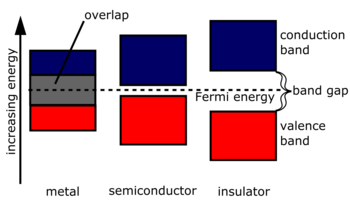# Energy band theory of solids1. WHAT IS A ENERGY BAND?

=> like energy levels, energy bands are also permitted "region" where a electron can exist in a atom or a molecule.  as the name suggests, energy band are much broader permitted regions for electrons. in a nutshell,an energy band is just combination of many energy levels with slightly different energies.

thus,  energy bands are permitted regions for a electron formed buy superposition of many similar energy levels with slightly different energies in a multi-atomic systems like soilds.

2. WHY ARE ENERGY BANDS  (formed) ?

=> in mono atomic system like gases (having no range order)i.e. where on atom doesnt affect another atom, there exist some energy levels where electrons are permitted.

but in a multi atomic system like solids(having a range order) i.e. where on atom affects another energy bands are formed. a nucleus of another atom in proximity can form another energy level with slightly differernt energy ie now there exist two energy levels of slightly differrnt energies. a third energy level is added with the presence of third atom in proximity. thus as the number of local atoms is high is soilds, a large number of energy level are formed with slightly diffrent energy forming a energy band.3. HOW DOES ENERGY BAND EXPALIN CONDUCTIVITY?

=> according to energy level theory the only electrons avilable for conduction are the electrons that and not bonded and in the outermost(valance) energy level.

=> but energy band theory states that conduction only occours when there are some electrons in the conduction band....but what is a conduction band?

=> conduction band is the outermost energy band in solids in which electrons are not bound to the nucleus by nay energy ie they are free to move. so conduction is determined by the presence of electrons in the conduction band.

=> but electrons are not readily avilable in the conduction band in all soilds. generally the outermost energy band in which the electrons are present without any thermal energy (at 0k) is called the valance band.

=> depending upon the nature of soilid there exist a energy gap between the conduction band and the valance band where electrons are forbidden. it is called a forbidden band and its bandwidth determineds the comnductivity of the solid.

4. CONDUCTORS, INSULATORS AND SEMICONDUCTORS.CONDUCTORS SEMICONDUCTORS INSULATORS the conduction band is filled at 0k the conduction band is empty at 0k the conduction band is empty at 0k the conduction band is filled at rooom temerature the conduction band is partially filled at room temperature the conduction band is empty at room temperature the forbiden energy band is absent the forbiden band is present the forbiden band is present band width of forbidden band = 0eV band width of forbidden band < 4eV band width of forbidden band > 4eV valance band and the conduction band are not distonct valance band and the conduction band are distonct valance band and the conduction band are distonct